# Compare Two Columns

To compare two columns, use IF, ISERROR and MATCH in Excel. You can display the duplicates or the unique values.

Before we start: do you want to compare two columns by highlighting the unique values in each column? Visit our page about comparing two lists.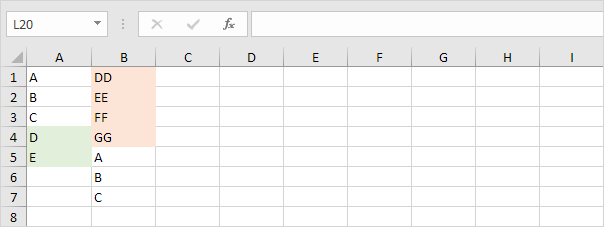### Display Duplicates

Let's start by comparing two columns and displaying the duplicates.

1. Display the duplicates in the first column (these values also occur in the second column).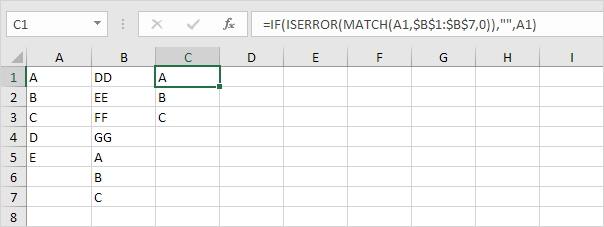Explanation: the MATCH function in cell C1 returns the number 5 (letter A found at position 5 in the range B1:B7). As a result, the ISERROR function returns FALSE and the IF function returns the value in cell A1. The MATCH function in cell C4 returns a #N/A error (no letter D in the range B1:B7). As a result, the ISERROR function returns TRUE and the IF function returns an empty string.

2. You can also display the duplicates in the second column.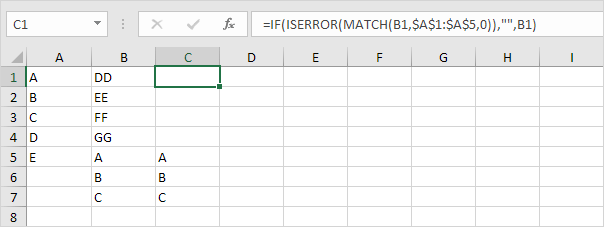Note: at step 1, we matched each value in the first column with the range in the second column. At step 2, we match each value in the second column with the range in the first column.

### Unique Values in Each Column

Do you want to compare two columns by displaying the unique values in each column? Simply swap the last 2 arguments of the IF function.

1. Display the unique values in the first column (these values do not occur in the second column).Note: take a look at the second picture on this page to see that we swapped the last 2 arguments of the IF function.

2. You can also display the unique values in the second column.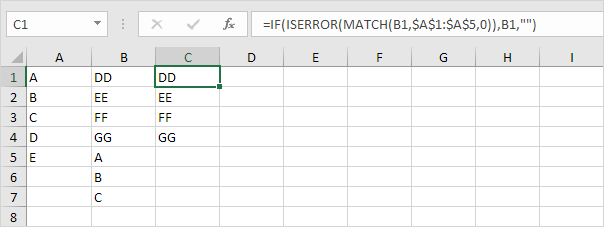Note: take a look at the third picture on this page to see that we swapped the last 2 arguments of the IF function.

### Row by Row Comparison

For a quick row by row comparison, simply use the IF function in Excel.

1. The IF function in cell C1 below returns Match because the value in cell A1 is equal to the value in cell B1.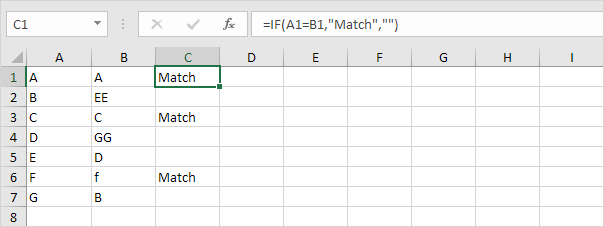2. To perform a case-sensitive comparison, add the EXACT function.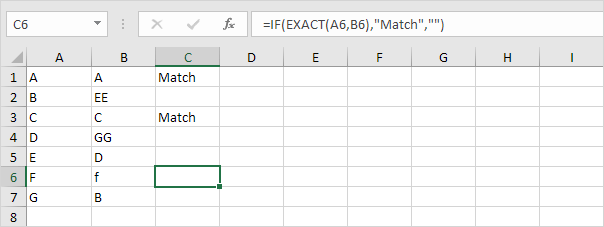3. Do you want to compare two columns by highlighting the differences in each row? Visit our page about Row Differences.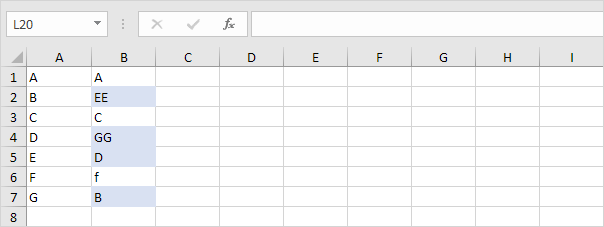Go to Next Chapter: Financial Functions